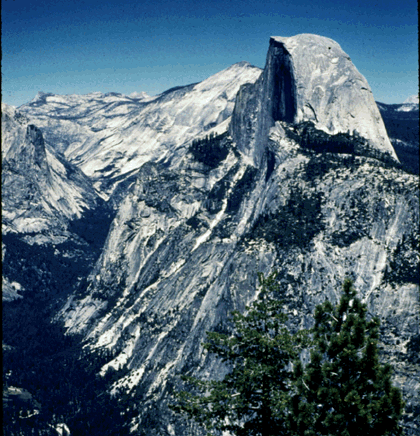## University of California, San Diego Physics 1b - Thermal Physics & Electromagnetism

 H. E. Smith Spring 2000Physics 1B - Tutorial #51. Half Dome in Yosemite National Park has a vertical north face which rises about 1450 m from the floor of Yosemite Valley.
• What is the gravitational potential difference (gravitational potential energy difference per unit mass) between Yosemite Valley and the top of Half Dome.
• What is the gravitational field near half dome.
• What is the potential gradient down the north face.
• During the summer there are hand railings which allow hikers to climb the south face of half dome. Using the picture above and a ruler or piece of graph paper, estimate the effective potential gradient and gravitational field down the south face.
• What do the lines of equipotential correspond to on a map of Yosemite Valley?2. Does a bird sitting on an uninsulated high voltage line get a shock? Explain.

3. Figure (a) below shows measurements of the electrical potential on a grid. Figure (b) shows equipotential contours.• Draw 50V, 70V & 100V contours in Fig. (a).
• If the gridlines in (a) are 1cm apart, estimate the electric field (magnitude and direction) at points A, B, C, & D.
• In Fig. (b) where is the electric field strongest?
• Where is it weakest?
• Suppose you were "pushing" a proton from the lowest contour to the highest contour. Which route would you take.
• Do you save energy by selecting one route over another.
• What happens to an electron which starts out at the 0V contour?

4. A very large conducting plate is charged with 1 C/m.
• What is the electric field above and below the plate?
• Draw a graph of the electric potential from below the plate to above the plate.
• What is the force on an electron 1 m above the plate?
A second plate, charged with -1 C/m is placed 1cm below the positively charged plate, parallel to it.
• What is the electric field below the lower plate? Between the plates? Above the upper plate.
• Draw a graph of the electric potential from below the lower plate to above the upper plate.
• Now what is the force on the electron?Physics 1B HomeTutorials

Gene Smith